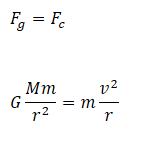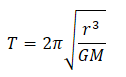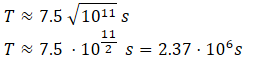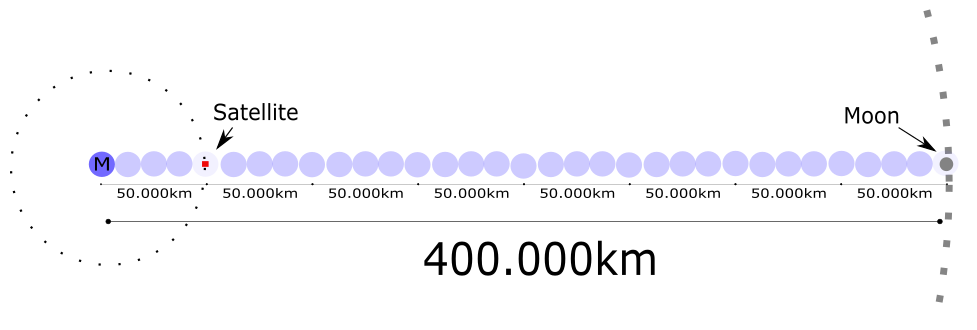top of page
Search

# Astronomy and Gravity TutorialKey Concepts:

Gravity

Kepler's Law

Circular Motion

Planetary Orbits

Question 1:

"The mass of the Earth, the mass of the moon and the distance between them are.

Determine the gravitational force of the moon upon the earth and the gravitational force of the earth upon the moon."

To solve this problem, we need:

1. A sketch of the situation we are trying to model.

2. An equation that tells us the gravitational force between two objects.

1. A sketch of the situation we are trying to model.Here we have defined the symbols:Now let us see what we can do about 2.

2. An equation that tells us the gravitational force between two objects.

In this situation, can we use Fg=mg and just plug in the mass of the moon or the earth? It is tempting, but the answer is NO! The gravitational acceleration, g=9.82 m/s^2, is only useful when we are close to the surface of the earth. It is only true in that very specific case. In general, we have to use Newton's Law of Gravitation:It basically says that:

"To find the gravitational force between two object, you have to multiply their masses, divide by the distance between them and multiply by a constant."

It makes sense that bigger masses means more force. It also makes sense that the further the masses get from each other, the weaker the force becomes. We can look up the Gravitational Constant, G, and plug in the numbers:That looks pretty complicated. Let us try to clean the expression up before we reach for the calculator. Move all the "raw numbers" to the left and all the "powers of 10" to the right:Much better! Let us take care of the powers of 10:Now for the "raw numbers" in front:So there is a pretty huge force between them! Notice that:

"Force on Earth from Moon = Force on Moon from Earth"

Question 2

"The use the information in Question 1 to determine how long it takes the moon to orbit the earth once."

Seriously? That seems downright impossible! But let us see what we would need in order to figure this out:

1. A sketch of the situation we are trying to model.

2. An equation that describes the force pulling the moon towards the earth.

3. An equation that describes the force pulling the moon away from the earth.

4. Some way of using this to figure out the orbit time of the moon.

1. A sketch of the situation we are trying to model.We have the moon going in a circular orbit around the earth. We know the radius of this orbit and we know the masses of the two planets. We want to find the time, T, it takes to go around the earth once.

Now on to 2.

2. An equation that describes the force pulling the moon towards the earth,

We need an equation that gives us the gravitational force between the two planets. We can just reuse:Now on to 3.:

3. An equation that describes the force pulling the moon away from the earth.

We know that the orbit is circular. If it is steady, then the centrifugal force pulling the moon away from the earth will be:Since we know that the moon is neither crashing into the earth or flying off into space, the two forces must be equal:Ok, that is nice, but how do we get the orbit time? The equation above only contains the speed of the moon! Well, we can say that it covers a distance of every time it spends an amount of time, T, going around.

Therefore the speed of the moon isTherefore:NICE! Now let us try to solve for T. Multiply by T on both sides:Cancel out the mass of the moon and multiply by r^2:Divide by GM:*Cough*ThisLooksALotLikeKepler'sThirdLaw*Cough* Oh, excuse me, I must have caught a cold. Anyways, let us now take the square root:Now let us plug in the actual values we have:Let us reuse the trick of moving all the "raw numbers" to the left, while keeping all the powers of 10 to the right:Let us calculate the "raw numbers" and take care of the powers of 10:Ok so how long is 2.37 million seconds anyways? Let us calculate how many days it corresponds to:Well, what do you know. It takes about a month for the moon to go around the earth. Who would have thought?

Question 3

"A satellite completes an orbit around the earth in 1 day. Determine the radius of its orbit."

Uhh, what? How on earth are we supposed to do that? Let us think about what we would need to tackle this problem:

1. A sketch of the system we are trying to model.

2. Some equation that relates the radius of the orbit to the time it takes to go around once.

Luckily, we can always make drawings, so let us do one for 1.Wait a second! This drawing is pretty much identical to the one we made for Question 2! In that question, we derived the following relation between orbital time and orbital radius:In Question 2, we assumed that the thing orbiting the earth was the moon, but the equation we derived doesn't really care about what specific object we are looking at. It just relates the orbit time of *any* object to the orbital radius of that object.

Conclusion: We can just reuse the formula from Question 2!

Let us try to solve for r^3 and then take the cube root:Great! Let us plug in some values. We need the orbit time in seconds, the Gravitational constant and the mass of the earth:Let us use the usual trick of collecting all the "raw numbers" to the left and all the powers of 10 to the right:Much better. Let us calculate:So the satellite orbits the earth at a radius of around 50.000 km. Recall that the moon orbits at:To put this into perspective, let us draw the orbits of the satellite and the moon to scale with the earth:Remember that the orbit of the satellite takes 1 day to complete, while the moon's orbit takes 27 days. Let us zoom out and see the full picture:Remember that one of those small blue circles is the size of the entire earth. The one in the middle is the actual earth that you are standing on right now.

In case you weren't aware: Space is HUGE!